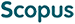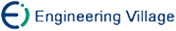姜纪远, 夏良, 章显, 陶卿. 一种具有O(1/T)收敛速率的稀疏随机算法[J]. 计算机研究与发展, 2014, 51(9): 1901-1910.
 引用本文: 姜纪远, 夏良, 章显, 陶卿. 一种具有O(1/T)收敛速率的稀疏随机算法[J]. 计算机研究与发展, 2014, 51(9): 1901-1910.Jiang Jiyuan, Xia Liang, Zhang Xian, Tao Qing. A Sparse Stochastic Algorithm with O(1/T) Convergence Rate[J]. Journal of Computer Research and Development, 2014, 51(9): 1901-1910.
 Citation: Jiang Jiyuan, Xia Liang, Zhang Xian, Tao Qing. A Sparse Stochastic Algorithm with O(1/T) Convergence Rate[J]. Journal of Computer Research and Development, 2014, 51(9): 1901-1910.## A Sparse Stochastic Algorithm with O(1/T) Convergence Rate

• 摘要: 随机梯度下降(stochastic gradient descent, SGD)是一种求解大规模优化问题的简单高效方法，近期的研究表明，在求解强凸优化问题时其收敛速率可通过α-suffix平均技巧得到有效的提升.但SGD属于黑箱方法，难以得到正则化优化问题所期望的实际结构效果.另一方面，COMID(composite objective mirror descent)是一种能保证L1正则化结构的稀疏随机算法，但对于强凸优化问题其收敛速率仅为O(logT/T).主要考虑“L1+Hinge”优化问题，首先引入L2强凸项将其转化为强凸优化问题，进而将COMID算法和α-suffix平均技巧结合得到L1MD-α算法.证明了L1MD-α具有O(1/T)的收敛速率，并且获得了比COMID更好的稀疏性.大规模数据库上的实验验证了理论分析的正确性和所提算法的有效性.

Abstract: Stochastic gradient descent (SGD) is a simple but efficient method for large-scale optimization problems. Recent researches have shown that its convergence rate can be effectively improved by using the so-called α-suffix averaging technique in solving the strongly convex problems. However, SGD is purely a black-box method, so it is difficult to obtain the expected effect on the learning structure while solving the regularized optimization problems. On the other hand, composite objective mirror descent (COMID) in the stochastic setting is a scalable algorithm which can effectively keep the sparsity imposed by L1 regularization；But it can only obtain an O(logT/T) convergence rate for the strongly convex optimization problems. In this paper, we focus on the generally convex optimization problem in the form of “L1 + Hinge”. To make full use of the α-suffix averaging technique, we first change it into a strongly convex optimization problem by adding an L2 strongly convex term. Then, we present an L1MD-α algorithm which combines the COMID algorithm and the α-suffix averaging technique. We prove that the L1MD-α algorithm can achieve an O(1/T) convergence rate. As a result, our L1MD-α algorithm not only obtains faster convergence rate but also better sparsity than COMID. Through extensive experiments on some typical large-scale datasets we finally verify the correctness of the theoretical analysis and effectiveness of the proposed algorithm./下载:  全尺寸图片 幻灯片
• 分享
• 用微信扫码二维码

分享至好友和朋友圈